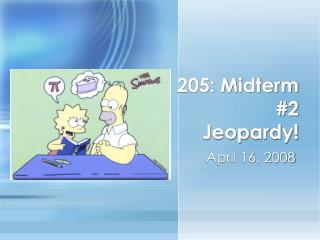DownloadDownload PresentationMath 205: Midterm #2 Jeopardy!

# Math 205: Midterm #2 Jeopardy!

Télécharger la présentation## Math 205: Midterm #2 Jeopardy!

- - - - - - - - - - - - - - - - - - - - - - - - - - - E N D - - - - - - - - - - - - - - - - - - - - - - - - - - -
##### Presentation Transcript

1. Math 205: Midterm #2Jeopardy! April 16, 2008

2. Jeopardy!

3. Definitions: 100 State Clairaut’s theorem. What part of the second derivative test uses this theorem? Back

4. Definitions: 200 State Fubini’s Theorem and how it applies to the following integral: Back

5. Definitions: 300 Suppose z = f(x,y) is a function in R3. If (a,b) is a point in the domain, describe the geometric meaning behind fx(a,b). Back

6. Definitions: 400 Define what it means for a function to be continuous at a point (a,b) and give a function in R3 that is discontinuous. Back

7. Partial Derivatives 1: 100 Compute the directional derivative of z = 2x2 - y3 at the point (0,1) in the direction of the vector u =2i- j. Back

8. Partial Derivatives 1: 200 Compute ∂z/∂t of z = 3xcosy, where x = 3st - t2 and y = s - 2sint. Back

9. Partial Derivatives 1: 300 Suppose you are at the point (0,π) a hill given by the function: z = 15 - x2 + cos(xy) - y2. If the positive y-axis represents north, and the positive x-axis represents east, what is your rate of ascent if you head northwest? Back

10. Partial Derivatives 1: 400 Find ∂z/∂y of the equation given by:zexz = y2 - yz. Back

11. Partial Derivatives 2: 100 Compute f of the function z = f(x,y) = 3x3/2y1/2. Back

12. Partial Derivatives 2: 200 Determine the tangent plane at the point (0, π/2) for the function z = 2cos(xy) - x2. Back

13. Partial Derivatives 2: 300 Find and classify all critical points of the function z = 1- 3x2 - y2 + 2xy. Back

14. Partial Derivatives 2: 400 Find and classify all critical points of the function z = 2xy-1 subject to the constraint x2 + y2 = 1. Back

15. Integration: 100 Reverse the order of integration in the following integral: Back

16. Integration: 200 Use polar coordinates to set up (but not evaluate) an integral to determine the volume under the sphere x2 + y2 + z2 = 4 within the first octant. Back

17. Integration: 300 Set up an integral to describe the area within the curve r = 2cos. Back

18. Integration: 400 Find the volume of the surface that lies under the cone z = 4 - √(x2+y2) and between the planes z = 1 and z = 2. Back